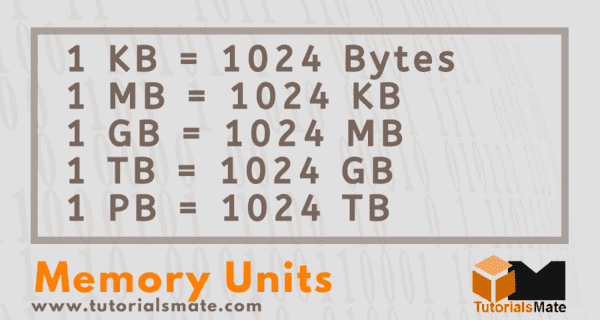# Memory Units of Computer: Bit, Byte, KB, MB, GB

Due to heavy usage and demand for computer systems, computer memory has also grown rapidly over time. Each generation of memory has brought a faster and higher capacity memory version of the computer. This has ultimately brought a new level of memory units and new terms for defining memory size.Therefore, it is necessary to know about all the memory units of the computer. Let us first understand what memory units are:

## What is a Memory Unit?

According to the definition, "A memory unit refers to the amount of memory/storage that is used to measure or represent data.

## Basic Memory Units in Computer

The following are the basic memory units in a computer system:

### Bit (Binary Digit)

A computer system uses electrical components (e.g., integrated circuits and semiconductors) to handle data or information stored in it. Such components can only understand and recognize the presence or absence of electrical signals. That is why a computer uses two different states, such as 0 (zero) and 1 (one).

The two symbols, 0 and 1, are known as bits or binary digits. While 0 indicates the absence (passive state) of the signal, 1 indicates the presence (active state) of the signal. However, a bit at a time can only store one of these two binary values, i.e., either 0 or 1. Therefore, multiple bits in a combined form are capable of holding a greater range of values.

### Nibble

A nibble is a collection of 4 bits.

### Byte

A byte is a collection of eight bits sequenced together to create a single computer alphabetical or numerical character. One byte, or eight bits, was the original amount of information needed to encode the characters of the text. The number was later standardized after upgrades in computer hardware.

A byte is referred to as the fundamental unit of measurement for the data on a computer system. It is because bytes can be more often represented in larger multiples, such as Kilobytes, Megabytes, Gigabytes, Terabytes, and Petabytes. Bytes are stored digitally (such as disk, tape, etc.) to define the amount of data on storage. Additionally, bytes also help in measuring memory and document size.

### Word

Like a byte, a computer word is also a combination of a fixed number of bits. It is another basic memory unit of a computer system. The number of bits in a word may vary for each computer system; however, it remains fixed for a particular computer.

Computer word length is commonly represented as word-size or word length. Because it varies from one system to another; therefore, it can be as short as 8 bits or as long as 96 bits. A computer system usually stores data or information in the form of computer words.

Note: Bits and bytes are known as basic building blocks of memory, where the bit is the smallest memory unit of a computer that is used to express data size. Additionally, a byte is the smallest unit that is used to represent data items or characters into higher memory units.

## Computer Memory Units Chart

The following table displays a list of all commonly used forms of computer memory units in ascending order:

Memory Unit Description
Kilobyte (KB) A kilobyte (1 KB) consists of 1024 bytes.
Megabyte (MB) A megabyte (1 MB) consists of 1024 kilobytes.
Gigabyte (GB) A gigabyte (1 GB) consists of 1024 megabytes.
Terabyte (TB) A terabyte (1 TB) consists of 1024 gigabytes.
Petabyte (PB) A petabyte (1 PB) consists of 1024 terabytes.

For simplicity and some technical reasons, the capacity of computer memory is usually expressed in multiples of power of two. This method eventually helped to express a very large number of bits and bytes easily.

## Summary

A memory unit is a method of expressing the capacity of memory or storage on a computer system. Although bits and bytes are basic forms of memory units, capacity is mainly expressed in terms (in multiples) of bytes.